Hostname: page-component-758b78586c-wkjwp Total loading time: 0 Render date: 2023-11-29T03:49:41.567Z Has data issue: false Feature Flags: { "corePageComponentGetUserInfoFromSharedSession": true, "coreDisableEcommerce": false, "useRatesEcommerce": true } hasContentIssue false

# Generalized Equivariant Cohomology and Stratifications

Published online by Cambridge University Press:  20 November 2018

## Abstract

Core share and HTML view are not possible as this article does not have html content. However, as you have access to this content, a full PDF is available via the ‘Save PDF’ action button.

For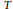$T$ a compact torus and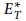$E_{T}^{*}$ a generalized$T$-equivariant cohomology theory, we provide a systematic framework for computing$E_{T}^{*}$ in the context of equivariantly stratified smooth complex projective varieties. This allows us to explicitly compute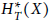$H_{T}^{*}\left( X \right)$ as an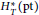$H_{T}^{*}\left( pt \right)$-module when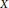$X$ is a direct limit of smooth complex projective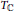${{T}_{\mathbb{C}}}$-varieties. We perform this computation on the affine Grassmannian of a complex semisimple group.

## Keywords

Type
Research Article
Information
Canadian Mathematical Bulletin , 01 September 2016 , pp. 483 - 496

## References

 Atiyah, M. F. and Bott, R., The Yang-Mills equations over Riemann surfaces.Philos. Trans. Roy. Soc. London. Ser. A 308(1983), no. 1505, 523615. http://dx.doi.org/10.1098/rsta.1 983.0017 Google Scholar
 Biatynicki-Birula, A.. Some theorems on actions of algebraic groups.Ann. of Math. 98(1973), 480497. http://dx.doi.org/10.2307/1 97091 5 Google Scholar
 Cole, M., C. Greenlees, J. P., and Kriz, I., The universality of equivariant complex bordism.Math. Z. 239(2002), no. 3, 455475. http://dx.doi.org/10.1007/s002090100315 Google Scholar
 Frenkel, E. and D. Ben-Zvi, Vertex algebras and algebraic curves. Second éd.,Mathematical Surveys and Monographs, 88, American Mathematical Society, Providence, RI, 2004. http://dx.doi.org/!0.1090/surv/088 Google Scholar
 Harada, M., Henriques, A., and Holm, T. S., Computation of generalized equivariantcohomologies ofKac-Moody flag varieties. Adv. Math. 197(2005), no. 1,198-221. http://dx.doi.org/10.101 6/j.aim.2 004.10.003 Google Scholar
 Hovey, M., Palmieri, J. H., and Strickland, N. P., Axiomatic stable homotopy theory.Mem.Amer. Math.Soc. 128(1997), no. 610.http://dx.doi.org/10.1090/memo/0610 Google Scholar
 Kamnitzer, J., Mirkovic-Vilonen cycles and polytopes. Ann. of Math.(2) 171(2010), no. 1, 245294. http://dx.doi.org/10.4007/annals.2010.171.245 Google Scholar
 Kirwan, E. C., Cohomology of quotients in symplectic and algebraic geometry. Mathematical Notes, 31, Princeton University Press, Princeton, NJ, 1984.http://dx.doi.org/10.1007/BF01145470 Google Scholar
 May, J. P., Equivarianthomotopy and cohomology theory. CBMS Regional Conference Series in Mathematics, 91, Published for the Conference Board of the Mathematical Sciences, Washington, DC; by the American Mathematical Society, Providence, RI, 1996. http://dx.doi.org/10.1090/cbms/091 Google Scholar
 Mirkovic, I. and Vilonen, K., Geometric Langlands duality and representations of algebraic groups over commutative rings. Ann. of Math.(2) 166(2007), no. 1, 95143. http://dx.doi.org/10.4007/annals.2007.1 66.95 Google Scholar
 Mitchell, S. A., Quillen's theorem on buildings and the loops on a symmetric space. Enseign.Math. (2) 34(1988), no. 1-2, 123166.Google Scholar
 Pressley, A. and Segal, G., Loop groups. Oxford Mathematical Monographs, Oxford Science Publications, The Clarendon Press, Oxford University Press, New York, 1986.Google Scholar
 Segal, G., Equivariant K-theory. Inst. Hautes Études Sci. Publ. Math. 34(1968), 129151.Google Scholar
 Sinha, D. P., Computations of complex equivariantbordism rings. Amer. J. Math. 123(2001), no. 4, 577605. http://dx.doi.org/10.1353/ajm.2001.0028 Google Scholar